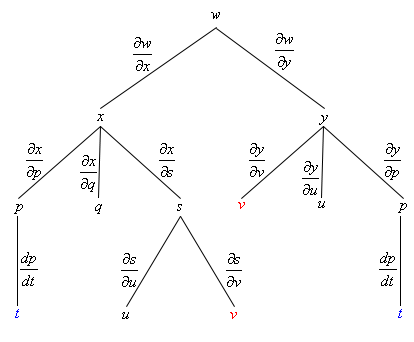Paul's Online Notes
Home / Calculus III / Partial Derivatives / Chain Rule
Show Mobile Notice Show All Notes Hide All Notes
Mobile Notice
You appear to be on a device with a "narrow" screen width (i.e. you are probably on a mobile phone). Due to the nature of the mathematics on this site it is best views in landscape mode. If your device is not in landscape mode many of the equations will run off the side of your device (should be able to scroll to see them) and some of the menu items will be cut off due to the narrow screen width.

### Section 13.6 : Chain Rule

7. Determine formulas for $$\displaystyle \frac{{\partial w}}{{\partial t}}$$ and $$\displaystyle \frac{{\partial w}}{{\partial v}}$$ for the following situation.

$w = w\left( {x,y} \right)\hspace{0.5in}x = x\left( {p,q,s} \right),\,\,\,\,y = y\left( {p,u,v} \right),\,\,\,\,s = s\left( {u,v} \right),\,\,\,\,p = p\left( t \right)$

Show All Steps Hide All Steps

Start Solution

To determine the formula for these derivatives we’ll need the following tree diagram.Some of these tree diagrams can get quite messy. We’ve colored the variables we’re interested in to try and make the branches we need to follow for each derivative a little clearer.

Show Step 2

Here are the formulas we’re being asked to find.

$\frac{{\partial w}}{{\partial t}} = \frac{{\partial w}}{{\partial x}}\frac{{\partial x}}{{\partial p}}\frac{{dp}}{{dt}} + \frac{{\partial w}}{{\partial y}}\frac{{\partial y}}{{\partial p}}\frac{{dp}}{{dt}}\hspace{0.5in}\frac{{\partial w}}{{\partial v}} = \frac{{\partial w}}{{\partial x}}\frac{{\partial x}}{{\partial s}}\frac{{\partial s}}{{\partial v}} + \frac{{\partial w}}{{\partial y}}\frac{{\partial y}}{{\partial v}}$